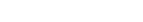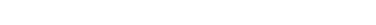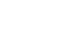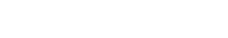# GMAT Math: Weighted Averages

Problems range from easy to hard.

1) On a ferry, there are 50 cars and 10 trucks.  The cars have an average mass of 1200 kg and the trucks have an average mass of 3000 kg.  What is the average mass of all 60 vehicles on the ferry?

(A) 1200 kg
(B) 1500 kg
(C) 1800 kg
(D) 2100 kg
(E) 2400 kg

2) In Plutarch Enterprises, 70% of the employees are marketers, 20% are engineers, and the rest are managers.  Marketers make an average salary of \$50,000 a year, and engineers make an average of \$80,000.  What is the average salary for managers if the average for all employees is also \$80,000?

(A) \$80,000
(B) \$130,000
(C) \$240,000
(D) \$290,000
(E) \$320,000

3) At Didymus Corporation, there are just two classes of employees: silver and gold.  The average salary of gold employees is \$56,000 higher than that of silver employees.  If there are 120 silver employees and 160 gold employees, then the average salary for the company is how much higher than the average salary for the silver employees?

(A) \$24,000
(B) \$28,000
(C) \$32,000
(D) \$36,000
(E) \$40,000

4) In a company of only 20 employees, 10 employees make \$80,000/yr, 6 employees make \$150,000/yr, and the 4 highest-paid employees all make the same amount.  If the average annual salary for the 20 employees is \$175,000/yr, then what is the annual salary of each highest-paid employee?

(A) \$250,000
(B) \$300,000
(C) \$350,000
(D) \$400,000
(E) \$450,000

5) In a certain apartment building, there are one-bedroom and two-bedroom apartments.  The rental prices of the apartment depend on a number of factors, but on average, two-bedroom apartments have higher rental prices than do one-bedroom apartments.  Let R be the average rental price for all apartments in the building.  If R is \$5,600 higher than the average rental price for all one-bedroom apartments, and if the average rental price for all two-bedroom apartments is \$10,400 higher that R, then what percentage of apartments in the building are two-bedroom apartments?

(A) 26%
(B) 35%
(C) 39%
(D) 42%
(E) 52%

6) At a certain upscale restaurant, there just two kinds of food items: entrees and appetizers.  Each entrée item costs \$30, and each appetizer item costs \$12.  Last year, it had a total of 15 food items on the menu, and the average price of a food item on its menu was \$18.  This year, it added more appetizer items, and the average price of a food item on its menu dropped to \$15.  How many appetizer items did it add this year?

(A) 3
(B) 6
(C) 9
(D) 12
(E) 15

Solutions will appear at the end of the article.

## Weighted average

For the purposes of the GMAT, the weighted average situations occurs when we combine groups of different sizes and different group averages.  For example, suppose in some parameter, suppose male employees in a company have one average score, the females have another average score, and gender non-binary/non-conforming employees (GNB/NC) have yet another average. If there were an equal number of men, women, and GNB/NC folks, we could just average the three separate gender averages: that would be ridiculously easy, which is precisely why the GMAT will never present you with groups of equal size in such a question.  Instead, the number of male, female, and GNB/NC employees will be profoundly different, one significantly outnumbering the other, and then we will have to combine the individual gender averages to produce a total average for all employees.  That’s a weighted average.

It is common for weighted average problems on the GMAT to have only two groups, but sometimes there are three (like the above problem), and conceivably, on a very hard problem, there could be four groups.  Each group is a different size, each has its own average, and the job is to find the average of everyone all together.  Or, perhaps the question will give us most of the info for the individual groups, and give us the total average for everyone, and then ask us to find the size or average of a particular group.

We have three basic approaches we can take to these question.

## Approach I: averages & sums

Remember that, even with ordinary average questions, thinking in terms of the sum can often be helpful.  We can’t add or subtract averages, but we can add or subtract sums! Right there is the key to one approach to the weighted average situation.  If we calculate the sums for each separate group, we can simply add these sums to get the sum of the whole group.  Alternately, if we know the size of the total group and the total average for everyone, we can figure out the total sum for everyone, and simply subtract the sums of the individual groups in order to find what we need.

Some weighted average problems give percents, not actual counts, of individual groups.  In that case, we could simply pick a convenient number for the size of the population, and use the sums method from there.  For example, if group A has 20% of the population,  group B has 40% of the population,  and group C also has 40% of the population,  then we could just pretend that group A has one person, groups B & C each have two people, and total population has five people.  From this, we could calculate all our sums.

This method always works, although is not always the most convenient in more advanced problems.  This will be demonstrated in a few of the answers below.

## Approach II: proportional & percentages

Sometimes the information about the sizes of individual groups is given, not in absolute counts of members, but simply in percents or proportions.  In the problems above, question #2 simply gives percents, and question #5 asks for a percent.   Yes, we could use Approach I, but there’s a faster way.

In Approach II, we simply multiply each group average by the percent of the population, expressed as a decimal, which that group occupies.  When we add these products up, the sum is magically the total average for everyone.  Suppose we have three groups, groups J and K and L which together constitute the entire population.  Suppose this summarizes their separate information:The percent have to be expressed in decimal form, so that:Then, the total average is simply given byThis approach will be demonstrated in #2 below.

## Approach III: proportional placement of total average

This final method can be hard to understand at first, but if you appreciate it, it is an incredibly fast time-saver.  This approach only works if there are exactly two groups, no more.

Suppose there are two groups in a population, group 1 and group 2, and suppose that group 1 is bigger than group 2.  Let’s also suppose that group 1 has a lower group average, and group 2 has the higher group average.  Of course, the total combined average of the two groups together will be between the two individual group averages.  In fact, because group 1 is bigger, the combined average will have to be closer to group 1’s average, and further away from group 2’s average.

Now, think about a number line, with the two individual group averages and the total averages indicated on the number line.On that number line, I have labeled d1, the distance from the average of group #1 to the combined average, and d2, the distance from the combined average to the average of group 2.  The ratio of these two distances is equal to a ratio of the size of the two individual groups.  Let’s think about this.  The bigger group, here group 1, will have more of an effect on the combined average and therefore will be closer to the combined average—a smaller distance.  Therefore, the ratio of the distances must equal the reciprocal of the same ratio of the sizes of the groups:Let’s say group 1 is 3 times larger than group 2.  This means d2, the distance from group 2’s average to the combined average, would have to be 3 times bigger than d1, the distance from group 1’s average to the combined average.  If the latter is x, then the former is 3x, and the total distance is 4x.  If we know the averages of the two groups individually, we would simply have to divide the difference between those group averages by 4: the combined average would be one part away from group 1’s average, or three parts away from group 2’s average.

This approach is hard to explain clearly in words.  You really have to see it demonstrated in the solutions below to understand it fully.   Once you understand it, though, this is an extremely fast method to solve many problems.

## Summary

If the above article gave you any insights, you may want to give the practice problems another look.  Remember, in your practice problems on weighted averages, practice all three of these methods.  The more ways you have to understand any problems, the more options you will have on test day!## Practice problem explanations

1) Method I: using sums

We will divide the two masses by 1000, 1.2 and 3 respectively, to simplify calculations.   Note the use of the Doubling and Halving trick in the first multiplication.

sum for cars = 50*1.2 = 100*0.6 = 60

sum for trucks = 10*3 = 30

total sum = 60 + 30 = 90

To find the total average, we need to divide this total sum by the total number of vehicles, 60.Since we divided masses by 1000 earlier, we need to multiply by 1000 to get the answer.  Total average = 1500 kg.  Answer = (B)

Method II: proportional placement of the total average

Cars to trucks is 5:1, so if the distance between the car’s average and truck’s average were divided into 6 parts, the car’s average is 1 part away from the total average, and the truck’s average is 5 parts away.

Well, the difference in the two group averages is 3000 – 1200 = 1800 kg.  Divide that by six: each “part” is 300 kg.  Well, the total average must be 300 kg bigger than 1200 kg, or 5*300 kg smaller than 3000 kg.  Either way, that’s 1500 kg.  Answer = (B)

2) We will approach this use the proportion & percents approach.  Divide all dollar amounts by 1000 for smaller numbers.  Multiply each group average by the percent expressed as a decimal:

marketers = 0.70*50 = 35

engineers = 0.20*80 = 16

managers = 0.10*x = 0.1x

where x is the average salary for the managers.  These three should add up to the average for all employees:

35 + 16 + 0.1x = 80

0.1x = 80 – 35 – 16

0.1x = 29

x = 290

Now, multiply the 1000 again, to get back to real dollar amounts.  The average salary for managers is \$290,000.  Answer = (D)

3) Method I: using sums

We can use this if we pick a value for the average salary for the silver employees.  It actually doesn’t matter what value we pick, because averages will fall in the same relative places regardless of whether all the individual values are slid up and down the number line.  The easiest value by far to pick is zero.  Let’s pretend that the silver folks make \$0, and the gold folks make \$56.  (I divided dollars by 1000 for simplicity).

Now, we also have to simplify the numbers of employees.  We could reduce the number of employees as long as we preserve the relative ratio.

silver : gold = 120:160 = 12:16 = 3:4

So everything would be the same if we just had 3 silver employees and 4 gold employees.  OK, now find the sums.

silver = 3*0 = 0

gold = 4*56

I am not even going to bother to multiply that out, because we know that the next step is to divide by 7, the total number of employees.

total average = 4*56/7 = 4*8 = 32

The average salary is \$32,000, which is \$32,000 higher than the average for the silver employees.  Answer = (C)

Method II: proportional placement of the total average

The ratio of silver employees to gold employees is

silver : gold = 120:160 = 12:16 = 3:4

If we divide the distance between the two averages by 7, then silver will be “four parts” away from the total average, and gold will be “three parts” away.

Well, the difference is \$56,000, so that divided by 7 is \$8000.  That’s one part.  Four parts would be \$32,000, which has to be the distance from the silver average to the total average.

4) We will approach this using sums.  The individual employee numbers are small.  We will divide all dollar amounts by 1000, for easier calculations.  Call the highest-paid employee salary x.  Then the sums are

lowest = 10*80 = 800

middle = 6*150 = 3*300 = 900

highest = 4x

Individual sums must add up to the total sum.

800 + 900 + 4x = 3500

4x = 1800

2x = 900

x = 450

The salary of each of those four highest paid employees is \$450,000.

5) This question is designed for an analysis involving proportional placement of the mean.  First, observe that R is much closer to the average for one-bedroom apartments, so there must be more one-bedroom apartments and fewer two-bedroom apartments.

The ratio of the distances to R is

5600:10400 = 56:104

Cancel a factor of 8 from both 56 and 104

56:104 = 7:13

One-bedroom apartments are “13 parts” of the building, and two-bedroom apartments are “7 parts.”  That’s a total of 7 + 13 = 20 parts in the building.  Two-bedroom apartments constitute 7/20 of the apartments in the building.  Since 1/20 = 5%, 7/20 = 35%.

6) Method I: using sums

First, last year.  Let x be the number of entrees.  Then (15 – x) is the number of appetizers.  The sums are:

entrees = 30x

appetizers = (15 – x)*12 = 12*15 – 12x = 6*30 – 12x = 180 – 12x

total = 15*18 = 30*9 = 270

Notice the use of the Doubling and Halving trick in the second and third lines.  The two individual sums should add up to the total sum.

30x + 180 – 12x = 270

18x = 90

x = 5

They start out with 5 entrees and 10 appetizers.

Let N be the number of appetizers added, so now there are 5 entrees and (10 + N) appetizers.  We need to solve for N.  Again, the sums:

entrees = 5*30 = 150

appetizers = (10 + N)*12 = 120 + 12N

total = (15 + N)*15 = 225 + 15N

Again, the two individual sums should add up to the total sum.

150 + 120 + 12N = 225 + 15N

270 = 225 + 3N

45 = 3N

15 = N

Method I was do-able, but we had to solve for many values.

Method II: proportional placement of the total average

Originally, the entrée price was 30 – 18 = 12 from the total average, and the appetizer price was 18 – 12 = 6.  This means there must have been twice as many appetizers as entrees.  Therefore , with 15 items, there must have been 10 appetizers and 5 entrees.

The number of entrees doesn’t change.  The average drops to \$15, so the distance from the entrée price is now 30 – 15 = 15, and the distance from the appetizer price is now 15 – 12 = 3.  That’s a 5-to-1 ratio, which means there must be 5x as many appetizers as entrees.  Since there still are 5 entrees, there must now be 25 appetizers, so 15 have been added.

•